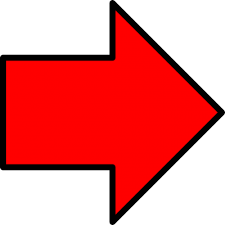HomeJAMBWAECNECONABTEBNEWS
Welcome To Examface.Net>>>>  The Nigeria's No 1 Exam Expo Website, With Many Years of Experience Verified by Google We Are The Best Among The Rest!.       ---

PAYMENT FOR WAEC & JAMB EXPO ARE ON!!!

---

---

--- ---

--- ---

---

MATHS SYMBOLS FOR OUR RUNS
MATHS SYMBOLS FOR OUR RUNS
This is how we send our Maths Symbols and the way you are suppose to write it on ur paper.

MATHS SYMBOLS

(1) / means ggdivision or divide
Examples:
2/2 means 2/2
2 whole no 3/4 means 2¾
M=wp+(1/3)yp^2 means m=wp + ¾y 2
(2) Log means logarithm
Example: (8) Log5 (base 8) means Log8 5
(3) * means multiplication
Example: 2*2 means 2×2
(4) ^ means Raise to power
Examples:
3^(-1) means 3 -1
5^(2) means 5 2
30cm^(-2) means 30cm -2
(5) Tita means θ
Example: Tan tita means tan θ
(6) Pie means π
Example: Pie R sqr h means πr 2 h
(7) Base means subscript
Example: 2 Base 3 means A 3
(8) Sqr root means
Examples:
√3-√6+2√3 means Root 3-root6+2root 3
√(¾) means sqr root(3/4)
(9) bar means a dash on top a number of letter
Example: X bar means x̅
(10) —(1) means equation 1
(11) Proportional means
Example: R is proportional to 3/4rut m means R ∝ ¾
(12) Alpha means α
(13) Beta means β
(14) Gamma means γ
(15) cube root means
(16) Mu means μ
(17) Rho means ρ
(18) Delta means δ
(19) Sigma means σ
(20) Tau means τ
(21) Ohm means Ω
(22) Lamda means λ
(23) Omega means ω
(24) Intersection means
Example: B intersection C means B∩C
(25) Union means U
Example: B union C means BUC
(26) Factorial means !
(27) complements in sets means
(28) (aq),(g),(l),(s) is used in chemistry to show the state of matter in equations
(29) Equivalent to means
(30) Not equal to means
(31) Quotation means “ ”
(32) Less or equal to means
(33) Greater or equal to
Example: (3/4)>=(1/2) means ¾ ≥ ½
(34) Plus or minus (or +-) means ±
Example: (3/4)<=(1/2) means ¾ ≤ ½

0 Response
FORUM POST LOCKED FOR COMMENTINGAds; Click Here Now to See How to Make Cool Cash Here in Examface.net

Contact Mr.Prof

best exam expo site / legit waec expo runz